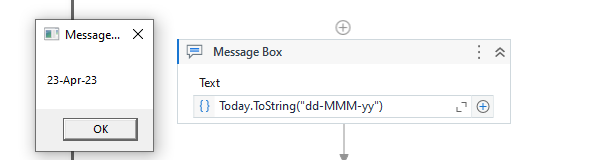# Condition - find/match data row-wise from excel

Hello all,

I have a data in excel with date-wise:

I want to write the if condition:
if current date is equal to the date value in the row i have to fill data in that column.

example : 4/23/2023 equals row (“4/23/2023”) fill data in the column (“4/23/2023”)

Thanking you

@Yugal_Raju

Method 1:

2. Then inplace of column name use `Now.ToString("MM/dd/yyyy 00:00:00")` …generally when reading data from excel the format would be like this for dates…so please use the date format accordingly if it is as seen in picture then use `dd-MMM-yy` eg: while filling in row 3 we can use `dt.Rows(3)(Now.ToString("MM/dd/yyyy 00:00:00") = "value to be filled"`

Method:”2:

1 Use find/replace activity and find the value `Now.ToString("dd-MMM-yy")` on the excel which will give the cell number as output
2. From the cell number we can get the column name and use the column value to fill the remaining cells Eg: if G1 is the output then we can use `Regex.Match(cellval,"[A-Za-z]*").Value` gives output as G

Hope this helps

Cheers

@Yugal_Raju

You can write as Today.ToString(“dd-MMM-yy”)

This result will be same as your column NameIf you want to compare the value then you place in a For Each Datatable and Inside If condition write as

CurrentRow(Today.ToString(“dd-MMM-yy”)).ToString